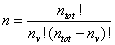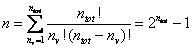Home News About Me Ph. D. Thesis Abstract Table of Contents 1. Introduction 2. Theory  Fundamentals of the Multivariate Data Analysis 2.1. Overview of the Multivariate Quantitative Data Analysis 2.2. Experimental Design 2.3. Data Preprocessing 2.4. Data Splitting and Validation 2.5. Calibration of Linear Relationships 2.6. Calibration of Nonlinear Relationships 2.7. Neural Networks  Universal Calibration Tools 2.8. Too Much Information Deteriorates Calibration 2.8.1. Overfitting, Underfitting and Model Complexity 2.8.2. Neural Networks and the Complexity Problem 2.8.3. Brute Force Variable Selection 2.8.4. Variable Selection by Stepwise Algorithms 2.8.5. Variable Selection by Genetic Algorithms 2.8.6. Variable Selection by Simulated Annealing 2.8.7. Variable Compression by Principal Component Analysis 2.8.8. Topology Optimization by Pruning Algorithms 2.8.9. Topology Optimization by Genetic Algorithms 2.8.10. Topology Optimization by Growing Neural Network Algorithms 2.9. Measures of Error and Validation 3. Theory  Quantification of the Refrigerants R22 and R134a: Part I 4. Experiments, Setups and Data Sets 5. Results  Kinetic Measurements 6. Results  Multivariate Calibrations 7. Results  Genetic Algorithm Framework 8. Results  Growing Neural Network Framework 9. Results  All Data Sets 10. Results  Various Aspects of the Frameworks and Measurements 11. Summary and Outlook 12. References 13. Acknowledgements Publications Research Tutorials Downloads and Links Contact Search Site Map Print this Page### 2.8.3.   Brute Force Variable Selection

The most obvious method of selecting a subset of variables is the examination of all combinations of variables. Thereby a subset of variables is selected, a neural network utilizing only these variables is calibrated, and the error of prediction of an independent test data set is calculated. Finally, the combination with the smallest error of prediction is chosen. Besides of some problems due to the random weight initialization of the networks and the limitation of the size of the data set, this so-called brute force variable selection is the most accurate approach.  However, this approach is only feasible for a very limited number of variables, as the number of variable subsets increases dramatically with the number of variables.

For a fixed number nv of variables to be selected from ntot variables in total, the number n of different variable subsets can be calculated as ,,:(14)

In the common case, when an optimal solution is searched, the number of variables to select is not fixed resulting in even more possible combinations n of variable subsets:(15)

For example 40 variables (refrigerant data introduced in section 4.5.1.1) result in 1 099 511 627 775 different combinations to be examined. If a fast up-to-date computer needs 1 minute for the training of a neural net (the time needed for the prediction can be neglected) the examination of all possible combinations needs 2 090 540 years computing time rendering the brute force variable selection useless for this work.

 Page 50 © Frank Dieterle, 03.03.2019